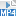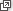## Content

The general form of a quadratic equation is $$ax^2+bx+c=0$$, where $$a,b,c$$ are given numbers with $$a \ne 0$$. These also arise naturally when solving real-world problems. For example, a ball thrown at an angle under gravity moves along a path that is parabolic. If we ask how far away the ball will land, then a quadratic equation arises.

There are a number of methods for solving quadratic equations. If the coefficients are all integers, the easiest and most common method involves factoring. But, as we have seen, it is not always possible or easy to factor a quadratic. So sometimes other techniques, such as completing the square or applying the quadratic formula, will need to be used. Students need to be comfortable and familiar with all these methods and be able to apply them strategically as needed.

When faced with a quadratic equation, we always put the equation into the standard form $$ax^2+bx+c=0$$.

#### Factoring method

The factoring method relies on the basic fact that if the product of two numbers is zero, then at least one of these numbers must be zero. That is, if $$AB=0$$, then $$A=0$$ or $$B=0$$. Hence, if we have a quadratic equation in which the quadratic has been factored, then we can equate each factor to 0 and solve the resulting linear equation. The method relies on being able to factor the quadratic easily, which is not always possible.

#### Example

Solve

$x^2+2x-15=0.$

#### Solution

\begin{gather*} \begin{aligned} x^2+2x-15 &= 0\\ (x+5)(x-3) &=0 \end{aligned}\\ \begin{aligned} x+5=0 &\text{ or } x-3=0\\ x=-5 &\text{ or } x=3. \end{aligned} \end{gather*}

The same procedure works for non-monic quadratics.

We may be given an equation which is a quadratic in disguise, that is, we can rearrange it into standard form to produce a quadratic or we can we make a simple substitution to turn it into a quadratic.

#### Example

Solve

$\frac{5x+7}{x-1}=3x+2.$

#### Solution

\begin{gather*} \begin{aligned} \frac{5x+7}{x-1}&= 3x+2\\ 5x+7&=(x-1)(3x+2)\\ 5x+7&= 3x^2-x-2\\ 3x^2-6x-9&=0\\ x^2-2x-3&=0\\ (x-3)(x+1)&=0 \end{aligned}\\ x=3 \text{ or } x =-1. \end{gather*}
##### Exercise 7

Solve

$4^x+2^{x+2}-32=0.$

Screencast of exercise 7#### Completing the square

In the event that the quadratic does not factor nicely, there may be irrational solutions or there may be no solutions. The method of completing the square will deal with both cases. It will, of course, also work even if the quadratic factors nicely. A detailed exposition of the method is in the TIMES module Quadratic equations (Years 9–10).

To complete the square on the equation $$x^2-4x-6=0$$, we take the $$-6$$ to the other side to produce $$x^2-4x=6$$. Now try to write the left-hand side as a perfect square by halving the coefficient of $$x$$. The square $$(x-2)^2$$ when expanded gives $$x^2-4x+4$$, so we add 4 to both sides of the original equation to produce $$(x-2)^2 = 6+4 = 10$$. We can now take the positive and negative square root and solve. Here is the full setting out:

\begin{gather*} \begin{aligned} x^2-4x-6 &=0\\ x^2-4x &=6\\ (x-2)^2 &= 6+4=10 \end{aligned}\\ \begin{aligned} x-2 = \sqrt{10}, &\qquad x-2 = -\sqrt{10}\\ x =2+\sqrt{10}, &\qquad x=2-\sqrt{10}. \end{aligned} \end{gather*}

The equation has two irrational solutions.

In the case of a quadratic with no real solutions, the method will lead to a square on the left-hand side and a negative number on the right. Thus, we cannot take the square root since all (real) squares are positive or zero. What will happen in the case when the quadratic has only one solution?

Example

Solve

$x^2+2x+7=0.$

#### Solution

\begin{align*} x^2+2x+7&=0\\ x^2+2x&=-7\\ (x+1)^2&=-7+1= -6. \end{align*}

Since the right-hand side is negative there is no solution.

If the quadratic is not monic, then you can divide both sides by the coefficient of $$x^2$$. This will produce fractions and in this case it is often better to resort to the quadratic formula.

With practice, the method of completing the square is often quicker than using the quadratic formula and the technique itself has many other applications in mathematics. For example, it is used to find the centre and radius of a circle whose equation is not given in standard form. It is also used in integration, and in higher mathematics to deal with partial fractions and Laplace transforms.

##### Exercise 8

The general equation of a circle with centre $$(a,b)$$ and radius $$r$$ is $$(x-a)^2+(y-b)^2=r^2$$. By completing the square on the terms with $$x$$ and then the terms with $$y$$, find the centre and radius of the circle

$x^2-2x+y^2-6y=1.$

Screencast of exercise 8#### The quadratic formula

The quadratic formula is simply a symbolic way of completing the square. That is, it gives a formula for the end result of applying the method of completing the square to a general quadratic equation $$ax^2+bx+c=0$$. To derive the formula, it is convenient to multiply the equation by $$4a$$ and do a slight variation on the completing the square method.

\begin{gather*} \begin{aligned} ax^2+bx+c &= 0\\ 4a^2x^2+4abx &= -4ac\\ (2ax+b)^2 &= b^2-4ac \end{aligned}\\ \begin{aligned} 2ax+b = \sqrt{b^2-4ac} &\quad\text{or}\quad 2ax+b = -\sqrt{b^2-4ac}\\ x = \frac{-b +\sqrt{b^2-4ac}}{2a} &\quad\text{or}\quad x= \frac{-b-\sqrt{b^2-4ac}}{2a}. \end{aligned} \end{gather*}

If the quantity $$b^2-4ac$$ is negative, then there are no solutions. This number is called the discriminant and often denoted by the Greek letter $$\Delta$$ (pronounced 'delta'). When solving quadratic equations it is generally a good idea to calculate the discriminant $$\Delta$$ first and think of the quadratic formula as

$x= \frac{-b+\sqrt{\Delta}}{2a} \quad\text{or}\quad x= \frac{-b -\sqrt{\Delta}}{2a}.$

What happens if $$\Delta = 0$$?

#### Example

Solve

$5x^2+3x-1=0.$

#### Solution

This equation does not appear to be amenable to factoring and although completing the square would work, it looks time consuming, since the quadratic is not monic. Hence we apply the quadratic formula.

The equation is $$5x^2+3x-1=0$$. Here $$a=5$$, $$b=3$$, $$c=-1$$ and so $$\Delta = 3^2-4\times 5 \times -1 = 29$$. Hence, the solutions are

$x=\frac{-3+\sqrt{29}}{10} \quad \text{or} \quad x= \frac{-3-\sqrt{29}}{10}.$

Next page - Content - Simultaneous linear equations